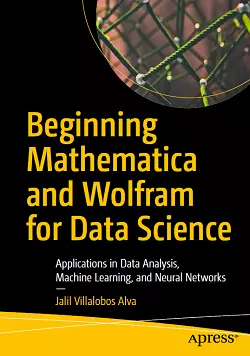# Beginning Mathematica and Wolfram for Data Science### eBook Details:

• Paperback: 439 pages
• Publisher: WOW! eBook (February 16, 2021)
• Language: English
• ISBN-10: 1484265939
• ISBN-13: 978-1484265932

### eBook Description:

Beginning Mathematica and Wolfram for Data Science: Applications in Data Analysis, Machine Learning, and Neural Networks: The first introduction to data science using Mathematica and Wolfram

Enhance your data science programming and analysis with the Wolfram programming language and Mathematica, an applied mathematical tools suite. The Beginning Mathematica and Wolfram for Data Science book will introduce you to the Wolfram programming language and its syntax, as well as the structure of Mathematica and its advantages and disadvantages.

You’ll see how to use the Wolfram language for data science from a theoretical and practical perspective. Learning this language makes your data science code better because it is very intuitive and comes with pre-existing functions that can provide a welcoming experience for those who use other programming languages.

What You Will Learn

• Use Mathematica to explore data and describe the concepts using Wolfram language commands
• Create datasets, work with data frames, and create tables
• Import, export, analyze, and visualize data
• Work with the Wolfram data repository
• Build reports on the analysis
• Use Mathematica for machine learning, with different algorithms, including linear, multiple, and logistic regression; decision trees; and data clustering

You’ll cover how to use Mathematica where data management and mathematical computations are needed. Along the way you’ll appreciate how Mathematica provides a complete integrated platform: it has a mixed syntax as a result of its symbolic and numerical calculations allowing it to carry out various processes without superfluous lines of code. You’ll learn to use its notebooks as a standard format, which also serves to create detailed reports of the processes carried out.